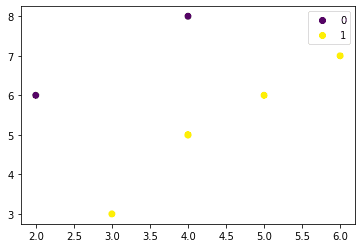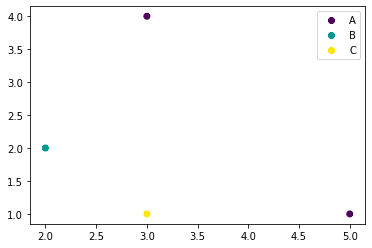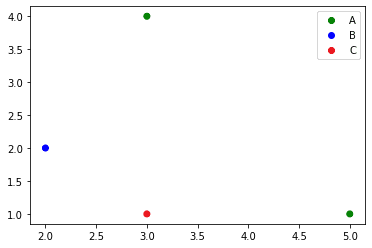search
Search
Map of Data Science
search toc
Thanks for the thanks!
close
account_circle
Profile
exit_to_app
Sign out
search
keyboard_voice
close
Searching Tips
Search for a recipe:
"Creating a table in MySQL"
Search for an API documentation: "@append"
Search for code: "!dataframe"
Apply a tag filter: "#python"
Useful Shortcuts
/ to open search panel
Esc to close search panel
to navigate between search results
d to clear all current filters
Enter to expand content previewDoc SearchCode Search BetaSORRY NOTHING FOUND!
mic
Start speaking...Voice search is only supported in Safari and Chrome.
Shrink
Navigate toMatplotlib
83 guides
keyboard_arrow_down
check_circle
Mark as learned
thumb_up
0
thumb_down
0
chat_bubble_outline
0
auto_stories new
settings

# Plotting scatter plot with category in Matplotlib

schedule Mar 5, 2023
Last updated
local_offer
PythonMatplotlib
Tags
map
Check out the interactive map of data science

# Basic example of plotting scatter plot with integer categories

Drawing a scatter plot when we have integer categories is simple:

``` import matplotlib.pyplot as pltys = [6,3,6,5,8,5,7]xs = [2,3,5,4,4,4,6]labels = [0,1,1,1,0,1,1]scatter = plt.scatter(xs, ys, c=labels)plt.legend(handles=scatter.legend_elements(), labels=[0,1])plt.show() ```

This generates the following plot:# Basic example of plotting scatter plot with string categories

To plot a scatter plot with string (non-integer) categories, use the following code:

``` import matplotlib.pyplot as pltimport pandas as pdlabels = ['A','B','A','C']classes = pd.Categorical(labels).codes # convert labels into array of integersscatter = plt.scatter([5,2,3,3], [1,2,4,1], c=classes)plt.legend(handles=scatter.legend_elements(), labels=labels)plt.show() ```

This results in the following plot:Here, we are first converting our string labels into numerical values using Pandas' `Categorical(~) `function:

``` classes = pd.Categorical(labels).codesclasses array([0, 1, 0, 2], dtype=int8) ```

# Plotting scatter plot with categories using custom colors

To plot a scatter plot with categories, use `ListedColormap`:

``` import matplotlib.pyplot as pltfrom matplotlib.colors import ListedColormapimport pandas as pdlabels = ['A','B','A','C']classes = pd.Categorical(labels).codescolours = ListedColormap(['g','blue','#EA131B'])scatter = plt.scatter([5,2,3,3], [1,2,4,1], c=classes, cmap=colours)plt.legend(handles=scatter.legend_elements(), labels=labels)plt.show() ```

This produces the following plot:thumb_up
thumb_down
Comment
Citation
Ask a question or leave a feedback...
thumb_up
0
thumb_down
0
chat_bubble_outline
0
settings
Enjoy our search
Hit / to insta-search docs and recipes!